I’ve read a few option books.
THANKS... This is probably the most comprehensive "greeks" article/book I’ve read.

Wonderful blog. …..
A wonder wealth of knowledge there. Thanks so much for your kindness in publishing it!

Thank you very much for the most concise and simplest option intro. Highly recommended.

So far, yours is the best blog/site on basic options notes in the web that I have chanced upon.

## Monday, November 7, 2011

### Behaviour of THETA in relation to TIME REMAINING TO EXPIRATION – With Past DATA and CHARTS

The following is the behavior of Theta in relation to Time to Expiration:

For ATM option, Theta increases as an option gets closer to the expiration date.
On the other hand, for ITM & OTM options, Theta decreases as an option is approaching expiration.
The above effects are particularly observed in the last few weeks (about 30 days) before expiration.

Using the same past actual data as shown in the previous post on the behavior of Delta, namely:
Options Chain for Call options of RIMM as at 3 Sep 2010, when the closing price is \$44.78 and Implied Volatility (IV) is 54.05, for expiration month of Sep 2010 (10 days to expiration), October 2010 (38 days to expiration) and Dec 2010 (101 days to expiration).

The following is the summary of Theta values for different Time to Expiration:For easier analysis, we can plot the Theta values of different Degree of Moneyness across various Time to Expiration, as follows:As can be seen from the table and the chart above:
For (near) ATM options (i.e. strike price \$45.00, because the stock price is \$44.78), Theta (in absolute value) is the higher for the options with expiration month “Sep-10” (nearer to expiration), as compared to “Oct-10” and “Dec-10”.
In other words, Theta (in absolute value) increases as time to expiration gets nearer.

Whereas for both deep ITM (strike price \$35.00 & \$37.50) and deep OTM options (strike price \$52.50 & \$55.00), Theta (in absolute value) is the lower for the options with expiration month “Sep-10” (nearer to expiration), as compared to “Oct-10” and “Dec-10”.
In other words, Theta (in absolute value) decreases as time to expiration gets nearer.

Note:
For Theta, we’re always comparing Theta here (whether it’s high or low) in terms of the absolute value, because the negative sign only represents the decaying effect.

Likewise, we’ll also compare Theta of different time to expiration at various strike prices, as shown in the chart below.As can be seen in the chart:
For all the three options with different time to expiration, Theta always behaves the same way, i.e. given the same time to expiration, Theta of ATM options is higher, and it gets lower as it moves towards deep ITM and deep OTM options.

That means:
Given the same time to expiration, ATM options will always decay faster as time goes by, as compared to deeper ITM and OTM options would.

However, the blue line (i.e. options with expiration month “Sep-10”) is much steeper than the red line (i.e. options with expiration month “Oct-10”) and green line (i.e. options with expiration month “Dec-10”).
This means:

Theta values for options with nearer time to expiration differ more significantly along various strike prices, as compared to those with further time to expiration.
The further the time to expiration is, the smaller the difference in the Theta values across different strike prices will be.

Conclusion:
Given the same time to expiration, ATM options will always decay faster as time goes by (i.e. have higher Theta) than the deeper ITM and OTM options would.

Given an ATM option, the option with nearer time to expiration will have the highest Theta (will decay the fastest), as compared to that with longer time to expiration.

Given a deeper ITM / OTM option, the option with nearer time to expiration will have the lowest Theta (will decay the slowest), as compared to that with longer time to expiration.

To view the list of all the series on the this topic, please refer to:
Behaviour of OPTION GREEKS in relation to TIME REMAINING TO EXPIRATION and IMPLIED VOLATILITY (IV) – With Past DATA and CHARTS.

Other Learning Resources:
* FREE Trading Educational Videos with Special Feature

Related Topics:
* Understanding Implied Volatility (IV)
* Understanding Option Greek
* Understanding Option’s Time Value
* Learning Candlestick Charts
* Options Trading Basic – Part 1
* Options Trading Basic – Part 2

## Saturday, October 22, 2011

### Behaviour of GAMMA in relation to TIME REMAINING TO EXPIRATION – With Past DATA and CHARTS

As discussed previously in the earlier post, here is the behavior of Gamma in relation to
Time to Expiration:

Assume all other factors unchanged:
For ATM options, Gamma increases (is higher) as time to expiration is nearing.
In contrast, for both deep ITM and deep OTM options, Gamma normally decreases (is lower) as time to expiration is nearing.

We will use the same past actual data as shown in the previous post on the behavior of Delta, namely:
Options Chain for Call options of RIMM as at 3 Sep 2010, when the closing price is \$44.78 and Implied Volatility (IV) is 54.05, for expiration month of Sep 2010 (10 days to expiration), October 2010 (38 days to expiration) and Dec 2010 (101 days to expiration).

Similarly, here is the summary of Gamma values for different Time to Expiration:As can be seen from the table, for both deep ITM (strike price \$35.00 & \$37.50) and deep OTM options (strike price \$52.50 & \$55.00), the Gamma values are the lowest for the options with expiration month “Sep-10” (nearer to expiration), followed by “Oct-10”, and then “Dec-10” (further to expiration).

On the other hand, for near ATM options (i.e. strike price \$45.00, because the stock price is \$44.78), the Gammas are the highest for the options with expiration month “Sep-10” (nearer to expiration), followed by “Oct-10”, and then “Dec-10” (further to expiration).

These prove the statement above.

Now, let’s compare Gamma of different time to expiration at various strike prices, as shown in the chart below.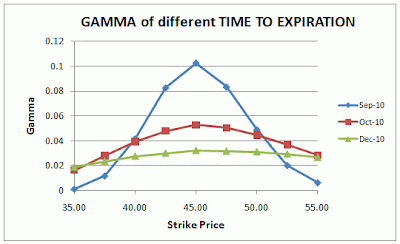As can be seen in the chart:

For all the three options with different time to expiration, Gamma always behaves the same way, i.e. Gamma of ATM options is always higher, and it gets lower as it moves towards deep ITM and deep OTM options.

That means:
Given the same time to expiration, the Delta of ATM options changes the most when the stock price moves up or down, as compared to deeper ITM and OTM options.

However, the blue line (i.e. options with expiration month “Sep-10”) is much steeper than the red line (i.e. options with expiration month “Oct-10”) and green line (i.e. options with expiration month “Dec-10”).
This shows that:

Gamma values for options with nearer time to expiration differ more significantly along various strike prices, as compared to those with further time to expiration.
The further the time to expiration is, the smaller the difference in the Gamma values across different strike prices will be.

Conclusion:
Given the same time to expiration, Gamma of ATM option will always be higher than Gamma of deeper ITM and OTM options.

Given an ATM option, the option with nearer time to expiration will have the highest Gamma, as compared to the option with longer time to expiration.

Given a deeper ITM or OTM option, the option with nearer time to expiration will have the lowest Gamma, as compared to the option with longer time to expiration.

To view the list of all the series on the this topic, please refer to:
Behaviour of OPTION GREEKS in relation to TIME REMAINING TO EXPIRATION and IMPLIED VOLATILITY (IV) – With Past DATA and CHARTS.

Other Learning Resources:
* FREE Trading Educational Videos with Special Feature

Related Topics:
* Understanding Implied Volatility (IV)
* Understanding Option Greek
* Understanding Option’s Time Value
* Learning Candlestick Charts
* Options Trading Basic – Part 1
* Options Trading Basic – Part 2

## Saturday, September 24, 2011

### Behaviour of DELTA in relation to TIME REMAINING TO EXPIRATION – With Past DATA and CHARTS

The following is the behavior of Delta in relation to Time to Expiration:

Assume all other factors unchanged:
As the time to expiration is nearing, the Delta of ITM options increases (i.e. ITM option’s Delta gets closer to 1 for Calls or to -1 for Puts) and the Delta of OTM options decreases (i.e. OTM option’s Delta gets closer to 0).

Now, let’s observe using the past real data.
The following is the Options Chain for Call options of RIMM as at 3 Sep 2010, when the closing price is \$44.78 and Implied Volatility (IV) is 54.05, for expiration month of Sep 2010 (10 days to expiration), October 2010 (38 days to expiration) and Dec 2010 (101 days to expiration).
(The rows highlighted in yellow are ITM options, while those in white are OTM).For easier reading and comparison, I summarize the Delta for different time to expiration as follow: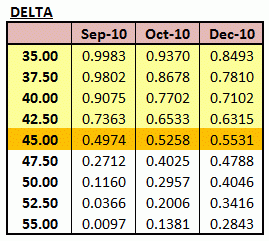As can be seen from the table, for ITM options (highlighted in yellow), the Deltas are the highest for the expiration month “Sep-10” (nearer to expiration), followed by “Oct-10”, and then “Dec-10” (further to expiration).

On the other hand, for OTM options, the Deltas are the lowest for the expiration month “Sep-10” (nearer to expiration), followed by “Oct-10”, and then “Dec-10” (further to expiration).

For near ATM options (i.e. the option’s strike price \$45.00, because the stock price is \$44.78), the Delta is about the same, i.e. close to 0.5.

These observations are in line with the statement above.

In addition, we can also look from different point of view, i.e. by comparing Delta at various strike prices at different time to expiration, as shown in the chart below.From the chart, we can see that:

The effect of stock price changes on the option price (i.e. Delta) are more “extreme“ for ITM and OTM options with nearer time to expiration, as compared to those with further time to expiration.
Nearer time to expiration will push the Deltas of ITM Calls closer to 1 (-1 for Puts) and the OTM option’s Delta closer to 0.

In contrast, for ATM options, the Delta is relatively unaffected to changes in time to expiration, i.e. all will have Deltas close to 0.5.

Implication
So, what’s the implication?
We can use this knowledge to help us consider and choose which options to use for trading, given the trading opportunities, expectation of whether the price movement is big or small, expected time frame, and options strategies.

For instance:
If you’re playing a swing trading and expect a stock’s price will change moderately within a short period, and you want to buy a straight Long Call to take advantage of this opportunity. In this case, you could consider using ITM options from a nearer time to expiration, as this option has higher Delta. Hence, when the stock price indeed increases as expected, you can gain more (in terms of dollar) from the increase in the option’s price.

However, given the scenario, suppose due to capital constraint, you would like to use OTM options, then choosing OTM options from a longer time to expiration should be better to take advantage from the stock price movement (in terms of dollar), as this option has higher Delta.
(Note: This is just a simple example about how to make use of the knowledge on Delta behavior in your trading. Actually, using OTM options in such case would have lower chance to be profitable, as an OTM option would require a very big increase in the stock price for the option to be profitable.)

The bottom line:
Whatever strategy you use, do consider the behaviors of the Option Greeks to help you choose which options to use (ITM, ATM, or OTM) to enhance the probability to make money.

Next, we’ll discuss about the behavior of the rest of the Options Greek.

To view the list of all the series on the this topic, please refer to:
Behaviour of OPTION GREEKS in relation to TIME REMAINING TO EXPIRATION and IMPLIED VOLATILITY (IV) – With Past DATA and CHARTS.

Other Learning Resources:
* FREE Trading Educational Videos with Special Feature

Related Topics:
* Understanding Implied Volatility (IV)
* Understanding Option Greek
* Understanding Option’s Time Value
* Learning Candlestick Charts
* Options Trading Basic – Part 1
* Options Trading Basic – Part 2

## Friday, September 23, 2011

### Behaviour of OPTION GREEKS in relation to TIME REMAINING TO EXPIRATION and IMPLIED VOLATILITY (IV) – With Past DATA and CHARTS

The past articles in this blog have discussed many times about the effect of time remaining to expiration and IV on Options Greeks.
In fact, not only this blog, many other websites have done the same too.
Nevertheless, generally these topics are only discussed qualitatively, as it is quite tedious and time consuming to show these using real data.

While there is an adage “A picture speaks a thousand words”, I am trying to show how Options Greeks behave in relation to the changes in time remaining to expiration or Implied Volatility (IV) by using the past real data and showing the relevant charts.

The following is the list of articles in this series:

Behaviour of Option Greeks in relation to TIME REMAINING TO EXPIRATION:
1. Delta
2. Gamma
3. Theta
4. Vega

Behaviour of Option Greeks in relation to IMPLIED VOLATILITY:
1. Delta
2. Gamma
3. Theta
4. Vega

By knowing better how Options Greeks behave in relation to the change in time remaining to expiration or Implied Volatility (IV), I hope this info can help you in your trading to enhance the probability to make money using whatever strategies that suit you.

Other Learning Resources:
* FREE Trading Educational Videos with Special Feature

Related Topics:
* Understanding Implied Volatility (IV)
* Understanding Option Greek
* Understanding Option’s Time Value
* Learning Candlestick Charts
* Options Trading Basic – Part 1
* Options Trading Basic – Part 2

## Sunday, September 11, 2011

### Historical Volatility – Part 7: Comparing HV

Go back to Part 6: Interpretation.

One other way to use the HV data is by comparing the values among different stocks, as well as for a particular stock.
Here are some of the possible ways and its purpose/use:

1) Comparing the HVs among different stocks.
Although the volatility always fluctuates, it tends to oscillate around some “normal” value over long period of time, which can be deemed as its “average” value. When the volatility is relatively high or low, it would then move back or reverse towards its average value.
Therefore, we can use the average value of HV to compare between the volatility of one stock with the other, in order to estimate whether the stock is relatively “more volatile” or “riskier” than the other.
A stock with higher HV is considered to be a “more volatile” or “riskier” stock than that with lower HV.

2) Comparing the HV of a particular stock a particular point of time with its own average HV value.
As mentioned earlier, the volatility of a stock will always keep fluctuating.
Comparing the HV of a particular stock a certain point of time with its own average HV value will allow us to what has happened to the stock price.
When the HV is high, that means the stock has been showing extreme fluctuations in price during the period.
When the HV is low, that means the stock has been in quiet or sideways trading during the period.

3) Comparing the HV of a particular stock in different period used for calculation.
Comparing the HV of a particular stock in different period can help to determine whether the volatility is rising or falling.
For example:
If the 30-day HV of a stock is 50% and 10-day HV of a stock is 15%, it suggests that the stock has recently experienced a sharp decline in volatility.

To view the list of all the series on “Historical Volatility”, please refer to: “More Understanding about HISTORICAL VOLATILITY

Other Learning Resources:
* FREE Trading Educational Videos with Special Feature

Related Topics:
* Understanding Implied Volatility (IV)
* Understanding Option Greek
* Understanding Option’s Time Value
* Learning Candlestick Charts
* Options Trading Basic – Part 1
* Options Trading Basic – Part 2

## Tuesday, August 23, 2011

### Historical Volatility – Part 6: Interpretation

Go back to Part 5: How To Annualise Standard Deviation.

After we know the definition and how to calculate HV, we’ll move on to its interpretation.

Example:
If it is known that the value of HV is 35%. Remember that this HV value is annualised, i.e. for one year.
As mentioned in the earlier post, assuming that price returns are normally distributed, about two-third of the time, an individual return would fall within one standard deviation of the mean, and about 95% of the time, an individual return would fall within two standard deviation of the mean.

That means, we can interpret that in one year, approximately two-thirds of the time, the stock returns would be between minus 35% and plus 35%. Or about 95% of the time, the stock return would be between minus 70% (= 2*35%) and plus 70%.

If the stock price is \$100, in one year, the price would probably be between \$65 and \$135 about two-thirds of the time. Or about 95% of the time, the stock price would be within \$30 to \$170 range.

Since the annualised HV is 35%, the estimated value of standard deviation for one month will be:That means, in one month, approximately two-thirds of the time, the stock returns would be between minus 10% and plus 10%. Or about 95% of the time, the stock return would be between minus 20% (= 2*10%) and plus 20%.

If the stock price is \$100, in one month, the price would probably be between \$90 and \$110 about two-thirds of the time. Or about 95% of the time, the stock price would be within \$80 to \$120 range.

With the annualised HV of35%, the estimated value of standard deviation for 10 days will be: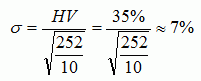That means, in 10 days time, approximately two-thirds of the time, the stock returns would be between minus 7% and plus 7%. Or about 95% of the time, the stock return would be between minus 14% (= 2*7%) and plus 14%.

If the stock price is \$100, in 10 days, the price is expected to be between \$93 and \$107 about two-thirds of the time. Or about 95% of the time, the stock price would be within \$86 to \$114 range.

Note:
Some people use the following formula to convert:This formula is actually the same as the above formula.
This formula is based on the one mentioned in Wikipedia, as discussed in the earlier post (Part 5).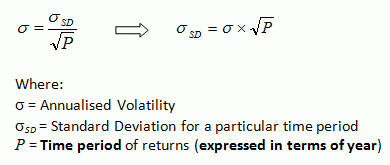Continue to Part 7: Comparing HV.

To view the list of all the series on “Historical Volatility”, please refer to: “More Understanding about HISTORICAL VOLATILITY

Other Learning Resources:
* FREE Trading Educational Videos with Special Feature

Related Topics:
* Understanding Implied Volatility (IV)
* Understanding Option Greek
* Understanding Option’s Time Value
* Learning Candlestick Charts
* Options Trading Basic – Part 1
* Options Trading Basic – Part 2

## Thursday, May 26, 2011

### Historical Volatility – Part 5: How To Annualise Standard Deviation

Go back to Part 4: Understanding Standard Deviation

As mentioned earlier, Historical Volatility is actually a standard deviation. The standard deviation can be calculated using historical price data in terms of daily, weekly, monthly, quarterly or yearly.
Historical Volatility is then expressed in terms of annualised standard deviation of % price returns, so that it can be compared across different stocks, regardless of the stock price and period used for HV calculation.

The formula to annualise the Standard Deviation (that may be calculated using either daily, weekly, monthly, quarterly or yearly) is as follow:Where:
HV = Historical Volatility (annualised)
Sigma = Standard Deviation for a particular time period
T = Number of times (count) of such time periods in a year

So, the value of T in the above formula will depend on the time period of the data used.

In the example used in Part 3, we use daily price returns to calculate standard deviation. Assuming there are 252 trading days in a year, the value of T = 252 / 1 day = 252, because there are 252 times of 1-day period in a year. Hence, we can annualise it by using the following formula: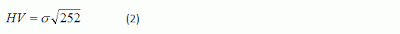Just for the sake of giving more examples for better understanding of the value of T.
Suppose that 3-day price return data (i.e. the closing prices for every 3 days) is used to calculate the standard deviation. In this case, the value of T = 252 / 3 days = 84, because there are 84 times of 3-day period in a year. Hence, the formula will be: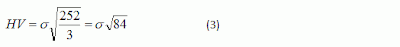In the case of monthly data is used (i.e. using month-end closing prices), the value of T will be 12 because there are 12 months in a year. Hence, the formula to annualise the monthly data is as follow: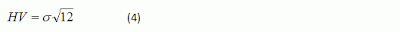For example:
If it is known that the “monthly” standard deviation of Stock ABC’s price returns is 15%, its Historical Volatility will be:Note:
In Wikipedia, the formula to annualise the standard deviation is as follow:
http://en.wikipedia.org/wiki/Volatility_(finance)Where:
Sigma = Annualised Volatility
Sigma SD = Standard Deviation for a particular time period
P = Time period of returns (expressed in terms of year)

To annualise a daily (i.e. 1 day) standard deviation, the value of P will be 1/252 (i.e. 1 day expressed in terms of year). So, the formula will be: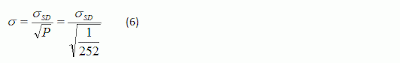This Formula (6) is actually the same as Formula (2), because:I found some people had commented that the formula in Wikipedia is not right. Actually, the formula is right. But we should understand what the logic is and understand the “definition” for the variables. Do compare the definition for T and P, and notice when we should multiply or divide when we want to annualise from daily standard deviation or to convert the annualised standard deviation into daily standard deviation. Just choose one that can make more sense to you.

Continue to Part 6: Interpretation

To view the list of all the series on “Historical Volatility”, please refer to: “More Understanding about HISTORICAL VOLATILITY

Other Learning Resources:
* FREE Trading Educational Videos with Special Feature

Related Topics:
* Understanding Implied Volatility (IV)
* Understanding Option Greek
* Understanding Option’s Time Value
* Learning Candlestick Charts
* Options Trading Basic – Part 1
* Options Trading Basic – Part 2

## Thursday, March 31, 2011

### Historical Volatility – Part 4: Understanding Standard Deviation

Go back to Part 3: Steps to Calculate HV using MS Excel (with Example)

As Historical Volatility (HV) is calculated using standard deviation, it might be good to understand better about the concept of standard deviation, so that we can interpret the meaning of HV better.

Standard deviation is a measure of data variability or dispersion (i.e. how spread out the data points from its mean).
When the standard deviation is low, that means the data points tend to be very close to its mean (i.e. the data is spread out over a small range of values).
When the standard deviation is high, that means the data points tend to be far away from its mean (i.e. the data is spread out over a large range of values).

This can be understood from the formula below as well: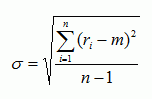The numerator in the formula is the summation of the difference between individual data point and the mean of the data set.

If the data points tend to be very close to its mean (less spread out from the mean value), the difference between each individual data point and the mean would be relatively small, and hence the summation of all differences and, in turn, the standard deviation will be small too.

On the other hand, if the data points tend to be far away from its mean (more spread out from the mean value), the difference between each individual data point and the mean would be bigger, and hence the summation of all differences and, in turn, the standard deviation will be big too.

In denominator, “n – 1” is used instead of “n” to get an unbiased estimator, because this standard deviation is derived based on sample, not population. (If the population is used, then the dominator will be “n”).
Since the standard deviation is estimated based on sample, using “n – 1” as the denominator will “inflate” the standard deviation value to “capture more risks” due to estimating the standard deviation based on sample only instead of population. (Remember that to estimate HV, we’ll never be able to use “population”).
This adjustment is particularly essential when we estimate the standard deviation based on a small number of observations (i.e. when n is relatively small). However, when n is big, the difference between using “n – 1” or “n” is not very significant.

Standard Deviation of Normal Distribution
One important attribute of the standard deviation is that in a Normal Distribution, about 66.8% (two third) of the data are within one standard deviation of the mean, and about 95% of the data are within two standard deviations of the mean.

In Historical Volatility, price returns are assumed to be normally distributed, like shown in the picture below.Source of picture: http://www.russell.com/us/glossary/analytics/standard_deviation.htm

Therefore, about two-third of the time, an individual return would fall within one standard deviation of the mean, and about 95% of the time, an individual return would fall within two standard deviation of the mean.

Continue to Part 5: How To Annualise Standard Deviation

To view the list of all the series on “Historical Volatility”, please refer to: “More Understanding about HISTORICAL VOLATILITY

Other Learning Resources:
* FREE Trading Educational Videos with Special Feature